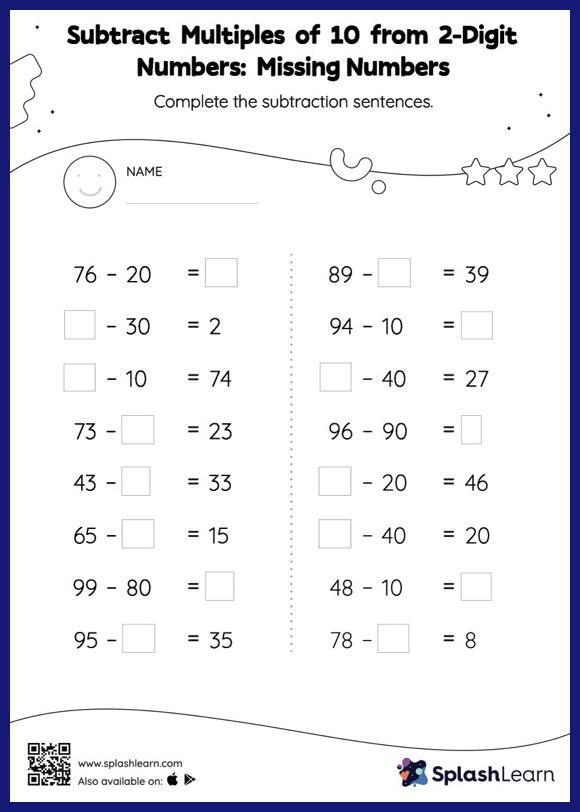# Subtract Multiples of 10 from 2-Digit Numbers: Missing Numbers Worksheet

Home > Subtract Multiples of 10 from 2-Digit Numbers: Missing NumbersTask your little mathematicians to crack the code of subtracting multiples of 10 from 2-digit numbers with this fun worksheet. If a minuend or subtrahend is missing, students use the count-back method or the relationship between addition and subtraction to determine the missing number. Also, they subtract the numbers if the missing number is the difference of the subtraction sentence. This subtract multiples of 10 from 2-digit numbers worksheet offers a tonne of practice with this idea. In each problem, the numbers are laid out in the horizontal format. Students should try to use different strategies involving composing and decomposing numbers to solve these problems. This will help them develop flexibility and fluency.